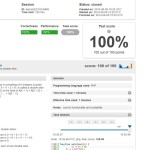# Peaks Demo Task at Codility.comCodility.com Tests 100/100 Solutions

Lesson 8 –  Prime and composite numbers – Peaks demo task solution code written in php. Gives 100/100 score at the time of publishing.

Solution in php:

```function solution(\$A) {
\$n = count(\$A);
if (\$n <= 2) {
return 0;
}
\$sum = array_fill(0,\$n,0);
\$last = -1;
\$D = 0;
\$sum = 0;
for (\$i = 1; \$i + 1 < \$n; ++\$i) {
\$sum[\$i] = \$sum[\$i - 1];
if ((\$A[\$i] > \$A[\$i - 1]) && (\$A[\$i] > \$A[\$i + 1])) {
\$D = max(\$D, \$i - \$last);
\$last = \$i;
++\$sum[\$i];
}

}
if ((\$sum[\$n - 1] = \$sum[\$n - 2]) == 0) {
return 0;
}
\$D = max(\$D, \$n - \$last);
for (\$i = (\$D >> 1) + 1; \$i < \$D; ++\$i) {
if (\$n % \$i == 0) {
\$last = 0;
for (\$j = \$i; \$j <= \$n; \$j += \$i) {
if (\$sum[\$j - 1] <= \$last) {
break;
}
\$last = \$sum[\$j - 1];
}
if (\$j > \$n) {
return \$n / \$i;
}
}
}
for (\$last = \$D; \$n % \$last; ++\$last){
;
}
return intval(\$n / \$last);
}
```

Given “AS IS”, can be ported from other languages from solutions found on the internet, please use with care.

Please note: we think that codility.com does not give a correct assessment of your real-world programming skills. For instance, reading the below would bring more understanding why: //x20x.co.uk/2014/02/why-i-refuse-to-use-codility-and-so-should-you/

This site uses Akismet to reduce spam. Learn how your comment data is processed.